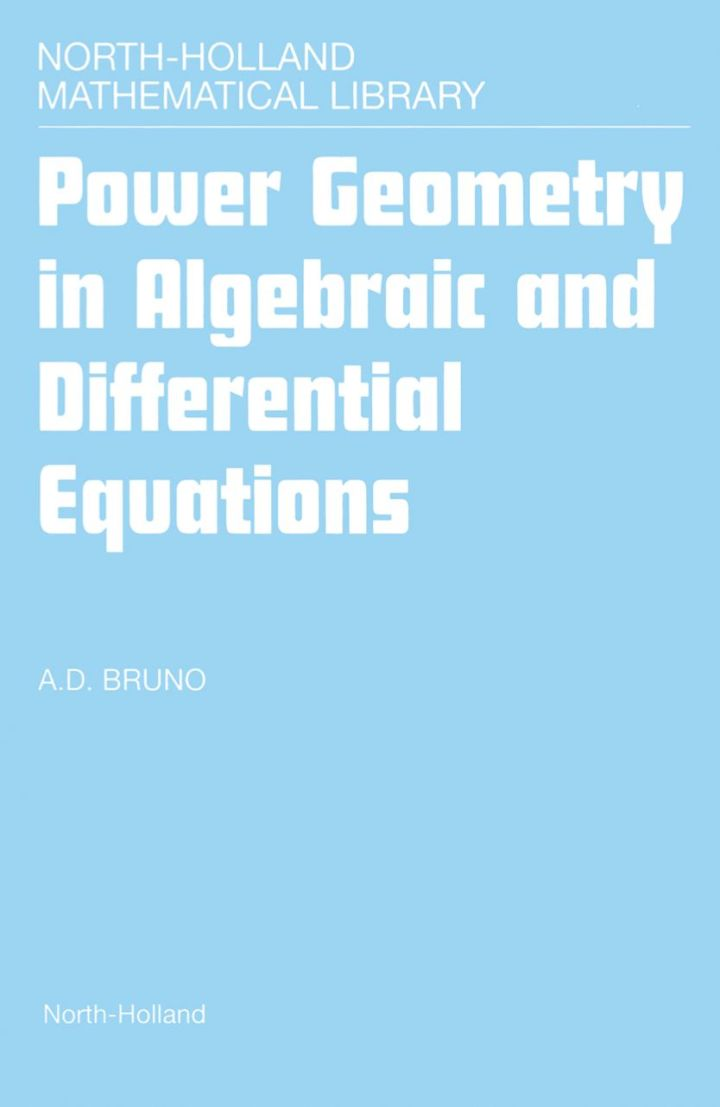# Power Geometry in Algebraic and Differential Equations

## Power Geometry in Algebraic and Differential Equations

By Bruno, A.D.

### Rent or Buy eTextbook

Expires on Apr 14th, 2022
\$175

Publisher List Price: \$179.99
Savings: \$4.99

The geometry of power exponents includes the Newton polyhedron, normal cones of its faces, power and logarithmic transformations. On the basis of the geometry universal algorithms for simplifications of systems of nonlinear equations (algebraic, ordinary differential and partial differential) were developed.
The algorithms form a new calculus which allows to make local and asymptotical analysis of solutions to those systems.
The efficiency of the calculus is demonstrated with regard to several complicated problems from Robotics, Celestial Mechanics, Hydrodynamics and Thermodynamics. The calculus also gives classical results obtained earlier intuitively and is an alternative to Algebraic Geometry, Differential Algebra, Lie group Analysis and Nonstandard Analysis.

Subject: Mathematics & Statistics -> Mathematics -> Mathematics GeneralPublisher: Elsevier S & T 08/2000
Imprint: Elsevier Science
Language: English
Length: 396 pages

ISBN 10: 0444502971
ISBN 13: 9780444502971
Print ISBN: 9780444502971

Live Chats